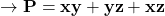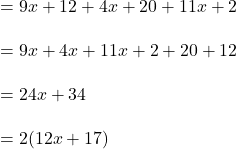## In the triangle xyz, zv is both the median and the altitude, if xy=9x+12 and xz=11x+2 and yz=4x+20 find the perimeter

Question

In the triangle xyz, zv is both the median and the altitude, if xy=9x+12 and xz=11x+2 and yz=4x+20 find the perimeter

in progress 0
5 months 2021-09-02T07:36:38+00:00 1 Answers 0 views 0